# SAT Math Multiple Choice Question 885: Answer and Explanation

### Test Information

Question: 885

15. If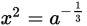, where x > 0 and a > 0, which of the following gives a in terms of x?

• A.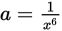• B.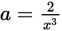• C.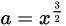• D.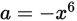Explanation:

A

Difficulty: Medium

Category: Passport to Advanced Math / Exponents

Strategic Advice: When a number (or a variable) is raised to a negative exponent, rewrite it as the reciprocal of the number with a positive exponent. You can also write fraction exponents as radicals to make using an inverse operation more recognizable.

Getting to the Answer: To write a in terms of x, you need to solve for a. To do this, you need to eliminate the fraction exponent, which also happens to be negative: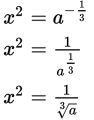To eliminate the radical (the cube root), cube both sides of the equation: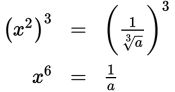Cross-multiply to get the a out of the denominator, and then divide both sides by x6: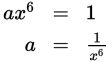Choice (A) is correct.